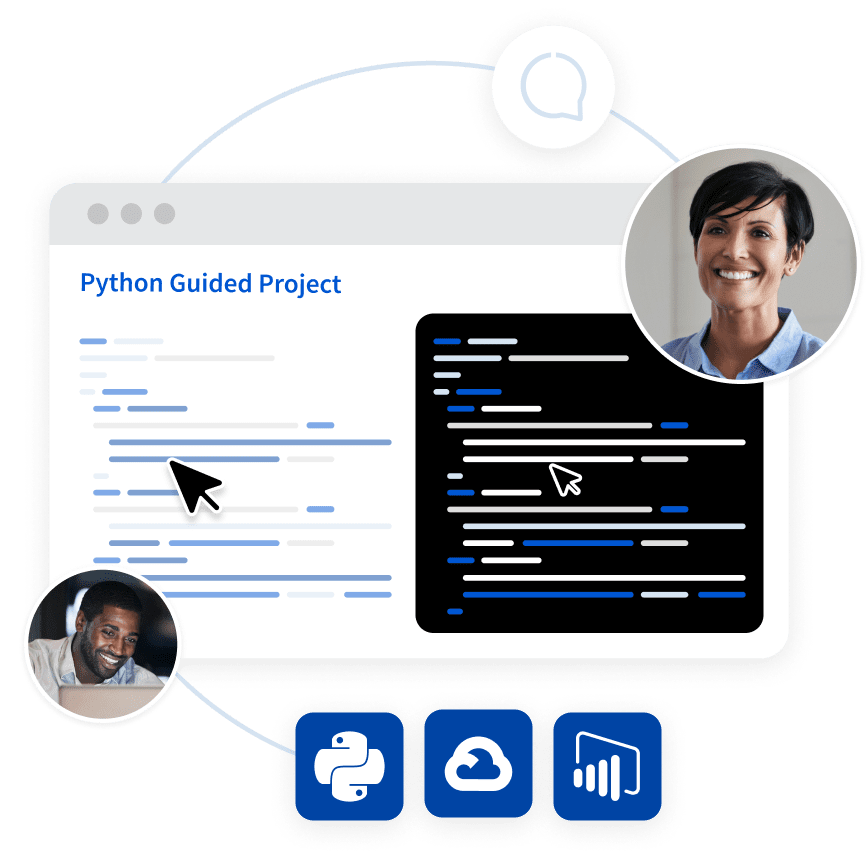## Python Tutorial

Python HOME Python Intro Python Get Started Python Syntax Python Comments Python Variables Python Data Types Python Numbers Python Casting Python Strings Python Booleans Python Operators Python Lists Python Tuples Python Sets Python Dictionaries Python If...Else Python While Loops Python For Loops Python Functions Python Lambda Python Arrays Python Classes/Objects Python Inheritance Python Iterators Python Scope Python Modules Python Dates Python Math Python JSON Python RegEx Python PIP Python Try...Except Python User Input Python String Formatting

## File Handling

Python File Handling Python Read Files Python Write/Create Files Python Delete Files

## Python Modules

NumPy Tutorial Pandas Tutorial SciPy Tutorial Django Tutorial

## Python Matplotlib

Matplotlib Intro Matplotlib Get Started Matplotlib Pyplot Matplotlib Plotting Matplotlib Markers Matplotlib Line Matplotlib Labels Matplotlib Grid Matplotlib Subplot Matplotlib Scatter Matplotlib Bars Matplotlib Histograms Matplotlib Pie Charts

## Machine Learning

Getting Started Mean Median Mode Standard Deviation Percentile Data Distribution Normal Data Distribution Scatter Plot Linear Regression Polynomial Regression Multiple Regression Scale Train/Test Decision Tree Confusion Matrix Hierarchical Clustering Logistic Regression Grid Search Categorical Data K-means Bootstrap Aggregation Cross Validation AUC - ROC Curve K-nearest neighbors

## Python MySQL

MySQL Get Started MySQL Create Database MySQL Create Table MySQL Insert MySQL Select MySQL Where MySQL Order By MySQL Delete MySQL Drop Table MySQL Update MySQL Limit MySQL Join

## Python MongoDB

MongoDB Get Started MongoDB Create Database MongoDB Create Collection MongoDB Insert MongoDB Find MongoDB Query MongoDB Sort MongoDB Delete MongoDB Drop Collection MongoDB Update MongoDB Limit

## Python Reference

Python Overview Python Built-in Functions Python String Methods Python List Methods Python Dictionary Methods Python Tuple Methods Python Set Methods Python File Methods Python Keywords Python Exceptions Python Glossary

## Module Reference

Random Module Requests Module Statistics Module Math Module cMath Module

## Python How To

Remove List Duplicates Reverse a String Add Two Numbers

## Python Examples

Python Examples Python Compiler Python Exercises Python Quiz Python Certificate

# Python JSON

JSON is a syntax for storing and exchanging data.

JSON is text, written with JavaScript object notation.

## JSON in Python

Python has a built-in package called `json`, which can be used to work with JSON data.

### Example

Import the json module:

import json

## Parse JSON - Convert from JSON to Python

If you have a JSON string, you can parse it by using the `json.loads()` method.

The result will be a Python dictionary.

### Example

Convert from JSON to Python:

import json

# some JSON:
x =  '{ "name":"John", "age":30, "city":"New York"}'

# parse x:

# the result is a Python dictionary:
print(y["age"])
Try it Yourself »

## Convert from Python to JSON

If you have a Python object, you can convert it into a JSON string by using the `json.dumps()` method.

### Example

Convert from Python to JSON:

import json

# a Python object (dict):
x = {
"name": "John",
"age": 30,
"city": "New York"
}

# convert into JSON:
y = json.dumps(x)

# the result is a JSON string:
print(y)
Try it Yourself »

## Learn to Filter Data in Python Like a Data AnalystTry a hands-on training sessions with step-by-step guidance from an expert. Try the guided project made in collaboration with Coursera now!

Get started

You can convert Python objects of the following types, into JSON strings:

• dict
• list
• tuple
• string
• int
• float
• True
• False
• None

### Example

Convert Python objects into JSON strings, and print the values:

import json

print(json.dumps({"name": "John", "age": 30}))
print(json.dumps(["apple", "bananas"]))
print(json.dumps(("apple", "bananas")))
print(json.dumps("hello"))
print(json.dumps(42))
print(json.dumps(31.76))
print(json.dumps(True))
print(json.dumps(False))
print(json.dumps(None))
Try it Yourself »

When you convert from Python to JSON, Python objects are converted into the JSON (JavaScript) equivalent:

Python JSON
dict Object
list Array
tuple Array
str String
int Number
float Number
True true
False false
None null

### Example

Convert a Python object containing all the legal data types:

import json

x = {
"name": "John",
"age": 30,
"married": True,
"divorced": False,
"children": ("Ann","Billy"),
"pets": None,
"cars": [
{"model": "BMW 230", "mpg": 27.5},
{"model": "Ford Edge", "mpg": 24.1}
]
}

print(json.dumps(x))
Try it Yourself »

## Format the Result

The example above prints a JSON string, but it is not very easy to read, with no indentations and line breaks.

The `json.dumps()` method has parameters to make it easier to read the result:

### Example

Use the `indent` parameter to define the numbers of indents:

json.dumps(x, indent=4)
Try it Yourself »

You can also define the separators, default value is (", ", ": "), which means using a comma and a space to separate each object, and a colon and a space to separate keys from values:

### Example

Use the `separators` parameter to change the default separator:

json.dumps(x, indent=4, separators=(". ", " = "))
Try it Yourself »

## Order the Result

The `json.dumps()` method has parameters to order the keys in the result:

### Example

Use the `sort_keys` parameter to specify if the result should be sorted or not:

json.dumps(x, indent=4, sort_keys=True)
Try it Yourself »